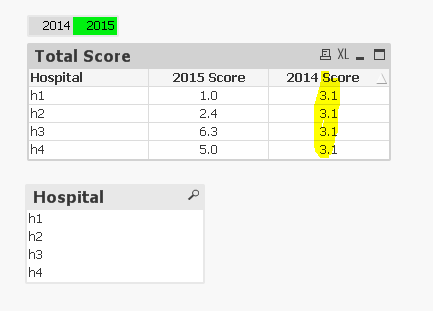QlikView App Dev

Discussion Board for collaboration related to QlikView App Development.

Announcements
QlikWorld 2022, LIVE in Denver CO., May 16-19, 2022. REGISTER NOW TO RECEIVE EARLY BIRD PRICING
cancel
Showing results for
Did you mean:Creator

When using Total, an expression shows same value for dimension.

Hi,

Please see my sample application attached.

I wanted to show both current and last year score for each hospital by simple calculation: Score= sum(Numerator * Weight)/sum(Weight) for all measures which a hospital has for the year. Because number of measures may vary each year, I used Total function to get all measures for last year score calculation. Now the expression is:

round(

sum(TOTAL {<Year={\$(=only(Year)-1)}>}Aggr(sum({<Year={\$(=only(Year)-1)}>} Numerator)*only({<Year={\$(=only(Year)-1)}>} Weight), MeasureID, Hospital))
/
Sum(TOTAL {<Year={\$(=only(Year)-1)}>}Aggr(Max({<Year={\$(=only(Year)-1)}>}Weight) , MeasureID,Hospital))
,0.1)

When I selected any hospital, the calculation is correct, if I don't select any, it defaults to all hospitals and gives me same result for all.If I select any hospital, the result is correct.I know my use of Total together with Aggr() must exist some problem, please help me to figure it out. Thanks a lot.

1 Solution

Accepted SolutionsMaster III

what about if you remove the 'TOTAL' from the expression?

like

round(

sum({<Year={\$(=only(Year)-1)}>}Aggr(sum({<Year={\$(=only(Year)-1)}>} Numerator)*only({<Year={\$(=only(Year)-1)}>} Weight), MeasureID, Hospital))

/

Sum({<Year={\$(=only(Year)-1)}>}Aggr(Max({<Year={\$(=only(Year)-1)}>}Weight) , MeasureID,Hospital))

,0.1)

5 RepliesMaster III

what about if you remove the 'TOTAL' from the expression?

like

round(

sum({<Year={\$(=only(Year)-1)}>}Aggr(sum({<Year={\$(=only(Year)-1)}>} Numerator)*only({<Year={\$(=only(Year)-1)}>} Weight), MeasureID, Hospital))

/

Sum({<Year={\$(=only(Year)-1)}>}Aggr(Max({<Year={\$(=only(Year)-1)}>}Weight) , MeasureID,Hospital))

,0.1)Master

round(sum(aggr(sum(aggr(sum({<Year={"\$(=only(Year)-1)"}>}Weight),Hospital,MeasureID))*sum(aggr(sum( {<Year={"\$(=only(Year)-1)"}>} Numerator),MeasureID,Hospital)),MeasureID,Hospital))
/
Sum(aggr(sum( {<Year={"\$(=only(Year)-1)"}>}Weight) , MeasureID,Hospital)),0.1)Creator
Author

Thanks for all of your help.Creator
Author